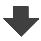Search:

# Reaction of hydrogen with copper(II)oxide

This reaction absorbes heat. How is such a reaction called?

It's an endothermic reaction.

What is the origin of the observed water?

The oxygen present in copper(II)oxide has combined to hydrogen.

Copper(II)oxide has lost its oxygen to be reduced to iron. What is that kind of reaction called?

It's a chemical reduction.

Formulate the equation !

$CuO$ $+$ $H_2$ $\longrightarrow$ $Cu$ $+$ $H_2O$.